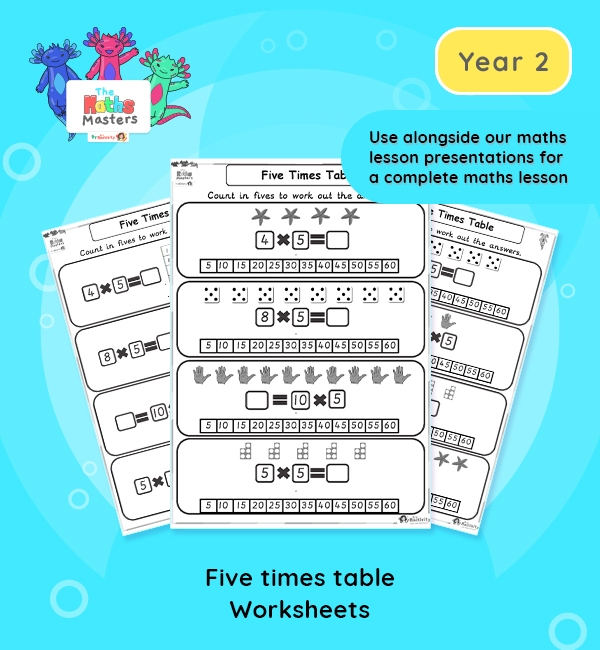# Year 2 | Five Times Table Worksheets## Year 2 multiplication worksheets

Aligned with the maths mastery approach, these differentiated Year 2 | Five Times Table Worksheets are designed to be used alongside our Year 2 | 5 Times Table Lesson Presentation for a complete maths lesson.  These worksheets require that children count in 5s to help them multiply by 5, with a picture prompt. The worksheets can also be cut up and used individually in children’s books to demonstrate knowledge and progression, without the need for a whole worksheet.

Topic: Multiplication and division

This lesson focuses on the five times table

Small Steps: 5 times table.

NC Links: Recall and use multiplication and division facts for the 2, 5, and 10 times tables. *Calculate mathematical statements for multiplication and division within the multiplication tables and write them using the multiplication, division and equals signs. *Solve problems involving multiplication and division using materials, arrays, repeated addition, mental methods and multiplication and division facts including problems in context. *Count in steps of 2, 3, 5, and 10.

TAF Statements: Working At  – Recall and use multiplication and division facts for 2, 5, and 10 and use them to solve simple problems.

Greater Depth – Use reasoning about number and relationships to solve more complex problems and explain their thinking.

Ready-to-progress criteria:  2MD-1 Recognise repeated addition contexts, representing them with multiplication equations and calculating the product within the 2, 5, and 10 multiplication tables.

Year 1 conceptual prerequisites: Count in multiples of 2, 5 and 10

Future applications: Use multiplication to represent repeated addition contexts for other group sizes.

## Recently Viewed